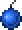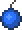•类型 \l!--\n --\g\{\{#vardefine:_typepage\!\{\{#or:\{\{l10n\!item_infobox\!typepage:@@@@\}\}\!\{\{tr\!\{\{ucfirst:@@@@\}\}\!link\ey\}\}\}\}\}\}\l!--\_\n --\g\{\{#vardefine:_typetext\!\{\{#or:\{\{l10n\!item_infobox\!type:@@@@\}\}\!\{\{tr\!\{\{ucfirst:@@@@\}\}\}\}\}\}\}\}\l!--\_\n --\g\{\{nowrap\!class\etag\!\{\{ifexists\!\{\{#var:_typepage\}\}\!\(\(\{\{#var:_typepage\}\}\!\{\{#var:_typetext\}\}\)\)\!\{\{#var:_typetext\}\}\}\}\}\}\l!--\n --\g\l!--\n --\g\{\{#vardefine:_typepage\!\{\{#or:\{\{l10n\!item_infobox\!typepage:@@@@\}\}\!\{\{tr\!\{\{ucfirst:@@@@\}\}\!link\ey\}\}\}\}\}\}\l!--\_\n --\g\{\{#vardefine:_typetext\!\{\{#or:\{\{l10n\!item_infobox\!type:@@@@\}\}\!\{\{tr\!\{\{ucfirst:@@@@\}\}\}\}\}\}\}\}\l!--\_\n --\g\{\{nowrap\!class\etag\!\{\{ifexists\!\{\{#var:_typepage\}\}\!\(\(\{\{#var:_typepage\}\}\!\{\{#var:_typetext\}\}\)\)\!\{\{#var:_typetext\}\}\}\}\}\}\l!--\n --\g 100 0（无击退力） ✔️ 25（快速度） 5 会摧毁大部分图格的小爆炸“投出去可不是件易事。” 99 / 501 需要 99 份

使用 \{\{#lstmap:\$soundfiles\$\!,\!%%%%\!\l!--\_print\_sounds\n --\g\lspan\_style\e"margin-left:2px;"\g\{\{sound\!\!\{\{trim\!%%%%\}\}\}\}\l/span\g\l!--\n --\g\!\}\} \{\{#lstmap:\$soundfiles\$\!,\!%%%%\!\l!--\_print\_sounds\n --\g\lspan\_style\e"margin-left:2px;"\g\{\{sound\!\!\{\{trim\!%%%%\}\}\}\}\l/span\g\l!--\n --\g\!\}\}

total: 1 row(s)

total: 1 row(s)

## 小贴士

• 粘性炸弹对于清理一个区域非常有用，因为他们比非粘性的对应物更加精确也更加安全。然而，它们整体覆盖范围较短。
• 因此，它们在试图摧毁猩红之心暗影珠时非常有用，因为玩家不需要设置障碍来防止它们滚走。
• 虽然粘性炸弹自身能卖 1，而其材料只能卖 61，因此相比而言，将炸弹制作成粘性炸弹比直接出售材料要更有利可图。